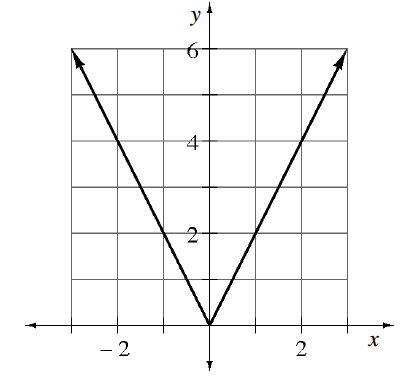### Home > PC3 > Chapter 2 > Lesson 2.1.2 > Problem2-22

2-22.

Is the function at below even, odd, or neither? Explain how you know by referencing the definition in problem 2-18.If the function is even, then $f(-1)=f(1)$.
If the function is odd, then $f(-1)=-f(1)$.